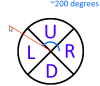# SOLVEDsprite_index in x and y axis change

#### kureoni

##### Member
thats the code

GML:
``````//for y axis

if(y > mouse_y){
sprite_index = spr_player_back
}

if(y < mouse_y){
sprite_index = spr_player_front
}

if(y != yprevious && y > mouse_y){
sprite_index = spr_player_walkback
}

if(y != yprevious && y < mouse_y){
sprite_index = spr_player_walkfront
}

if(y < mouse_y && x != xprevious){
sprite_index = spr_player_walkfront
}

if(y > mouse_y && x != xprevious){
sprite_index = spr_player_walkback
}

//for x axis

if(x < mouse_x && x = xprevious){
image_xscale = 3
sprite_index = spr_player
}

if(x > mouse_x && x = xprevious){
image_xscale = -3
sprite_index = spr_player
}

if(x < mouse_x && x != xprevious){
image_xscale = 3
sprite_index = spr_player_walk
}

if(x > mouse_x && x != xprevious){
image_xscale = -3
sprite_index = spr_player_walk
}``````
they work separately but they dont work together
any ideas?

its a topdown game

the character should walk in any direction facing the mouse position

example: if the player is walking upwards and the mouse is downwards, he should walk up facing down.

HELP

#### aristhemage

##### Member
If they work separately, then use them separately?

#### kureoni

##### Member
If they work separately, then use them separately?
one will only work if the other does not exist anywhere

#### BattleRifle BR55

##### Member
Instead of presenting a case for each scenario, group them. First, check for movement, then check for direction.

•kureoni

#### kureoni

##### Member
Instead of presenting a case for each scenario, group them. First, check for movement, then check for direction.
ill try

#### kureoni

##### Member
Instead of presenting a case for each scenario, group them. First, check for movement, then check for direction.
it wont work im gonna fcking kick my pc#### BattleRifle BR55

##### Member
Post what you tried.

#### EPonyA

##### Member
Looking at the code, it seems very confusing to me what you want. Here's what's happening:

1. The y-axis code determines whether the character should face up or down (in ALL cases where y != mouse_y), and whether it should be walking or not.
2. The x-axis code overwrites the sprite in ALL cases where x != mouse_x.

x == mouse_x is an edge case, so it nearly always overwrites sprite_index. But I think your entire approach to this problem is off-track, so far.

I've mocked up a super cheap diagram to help illustrate my point:(FYI, I was literally sleep deprived when I said that was 200 degrees lol)
Imagine a circle at the origin of your object. It has four quadrants right, up, left, and down.
R: 315 - 45
U: 45 - 135
L: 135 - 225
D: 225 - 315

Those values are the range of degrees that determine which quadrant any given angle is in. Now imagine an angle that points from the origin to your mouse co-ordinates ( angle = point_direction( x, y, mouse_x, mouse_y ).

If you do a little trick to keep that within the bounds of 0-360 exclusive, which is simply: angle = ( angle + 360 ) % 360;
Then, by running it through your known degree bounds of the quadrants, you can determine which quadrant the angle is pointing in!

Here's some GML:
GML:
``````var angle = point_direction(x, y, mouse_x, mouse_y);
angle = ( angle + 360 ) % 360; // Prevents a value of 360.

var walking = false;
if x != xprevious || y != yprevious {
walking = true;
}

if angle > 315 || walking <= 45 {
image_xscale = 3;

if walking {
sprite_index = spr_player_walk;
} else {
sprite_index = spr_player;
}
} else if angle > 45 && angle <= 135 {
if walking {
sprite_index = spr_player_walkback;
} else {
sprite_index = spr_player_back;
}
} else if angle > 135 && angle <= 225 {
image_xscale = -3;

if walking {
sprite_index = spr_player_walk;
} else {
sprite_index = spr_player;
}
} else if angle > 225 && angle <= 315 {
if walking {
sprite_index = spr_player_walkfront;
} else {
sprite_index = spr_player_front;
}
}``````
I hope this helps!

Last edited:

#### kureoni

##### Member
thanks for the help every1
there may be something else wrong
but that is fine, i gave up on the mouse position thingy etc
maybe later ill find that out